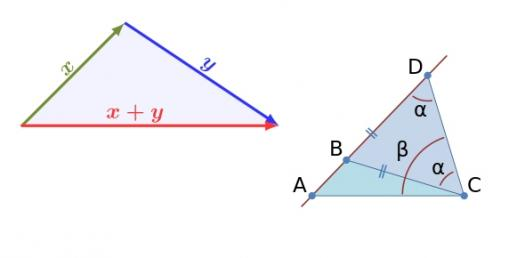# Triangle Inequality & Congruent Triangles Theorems

12 Questions | Total Attempts: 273SettingsThis will count as DOM # 1: Triangle Congruence.  You MUST show your final score to either Ms. A or Ms. MeGahee.  If you do not show either of us the score, you will receive a 0!  Good luck! You may use your notes / book.

• 1.
Which combination of congruent corresponding parts can you not use to prove two triangles congruent?
• A.

SAA

• B.

AAA

• C.

ASA

• D.

SAS

• E.

SSS

• 2.
Refer to the figure. Complete the congruence statement
• A.

Triangle VTU

• B.

Triangle TUV

• C.

Triangle VUT

• D.

Triangle UTV

• 3.
Two triangles are necessarily congruent if and only if __________.
• A.

Their corresponding angles are congruent.

• B.

Their corresponding sides and corresponding angles are congruent, and they are rotated to the same position.

• C.

Their corresponding sides and corresponding angles are congruent.

• D.

Two of their sides are congruent.

• 4.
by the _______________.
• A.

SAS Postulate

• B.

SSA Postulate

• C.

SSS Postulate

• D.

ASA Postulate

• 5.
• A.

SAS Postulate

• B.

ASA Postulate

• C.

AAA Postulate

• D.

SSS Postulate

• 6.
Which postulate or theorem shows that
• A.

ASA Postulate

• B.

SAS Postulate

• C.

SSS Postulate

• D.

AAS Theorem

• 7.
ΔSAM≅ΔRATWhich statement is NOT necessarily true?
• A.

MA = TA

• B.

MS = TR

• C.

• D.

• 8.
By which reason can it be proven that triangles DAB and DAC are congruent?
• A.

AAA

• B.

AAS

• C.

SSA

• D.

SSS

• 9.
Use the triangle inequality to determine the smallest angle in the figure.∠SCI
• A.

• B.

• C.

• D.

• 10.
Which pair of triangles would you use ASA to prove the congruence of the 2 triangles?
• A.

A

• B.

B

• C.

C

• D.

D

• 11.
List the sides of the triangle in order from SHORTEST to LONGEST.
• A.

BA, BC, AC

• 12.
The shortest side of ΔDEF is ___________.
Related TopicsBack to top